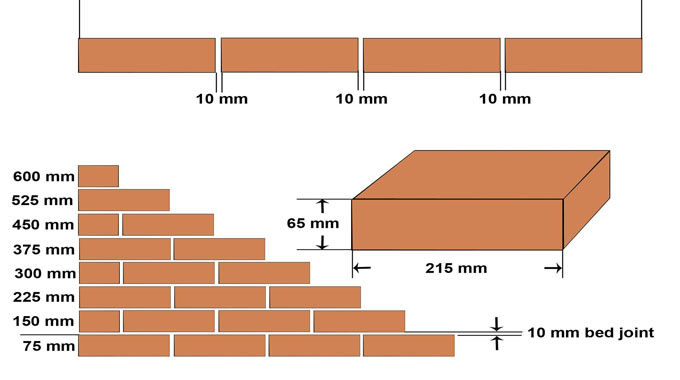# Deductions for openings in masonryThe following types of deductions are commonly found in masonry constructions.

### Rectangular Openings

For rectangular openings full deduction will be as follow :-

Deduction = Length x Height x Thickness of wall

### Segmental Arch Openings

With the existence of segmental arch opening over the rectangular opening, deduction in masonry is provided for both the rectangular opening & the segmental arch opening.

Area of rectangular part = L x H
Area of segmental part = 2/3L x R x R3 /2L

So, the total deduction is as follow :-

[L x H + 2/3L x R] x thickness of wall

### Semi-circular arch opening :

Semi-circular arch opening :
Area of semi-circular portion = πR2/2

But the approximate area of the semi circular section is as follow :-

= ¾ L x R
So, the total deduction
= [L x H + ¾ L x R] x thickness of wall

### Masonry of arch

The quantity of masonry in arch is calculated with cubic meter as an individual thing.

The quantity of arch masonry is subtracted from the total masonry.

Masonry of arch
= Centre length of arch x width of the arch x thickness of arch
So, the deduction for arch in masonry
= Lm x b x t

### Lintel over opening

An RCC lintel is constructed over the door or window opening.

The quantity of RCC of lintel is subtracted from the masonry work.

A bearing of 10 to 15 cm from the edge of opening is arranged to the lintel on either end of the opening. So,

Length of lintel (L) = Span of opening + 2 x bearing deduction for lintel = L x Width of wall x thickness of lintel.

Area of semi-circular portion = πR2/2

But the approximate area of the semi circular section is as follow :-

= ¾ L x R So, the total deduction
= [L x H + ¾ L x R] x thickness of wall

### Masonry of arch

The quantity of masonry in arch is calculated with cubic meter as an individual thing.

The quantity of arch masonry is subtracted from the total masonry.

### Masonry of arch

= Centre length of arch x width of the arch x thickness of arch
So, the deduction for arch in masonry
= Lm x b x t

### Lintel over opening

An RCC lintel is constructed over the door or window opening.

The quantity of RCC of lintel is subtracted from the masonry work.

A bearing of 10 to 15 cm from the edge of opening is arranged to the lintel on either end of the opening. So,

Length of lintel (L) = Span of opening + 2 x bearing deduction for lintel = L x Width of wall x thickness of lintel.